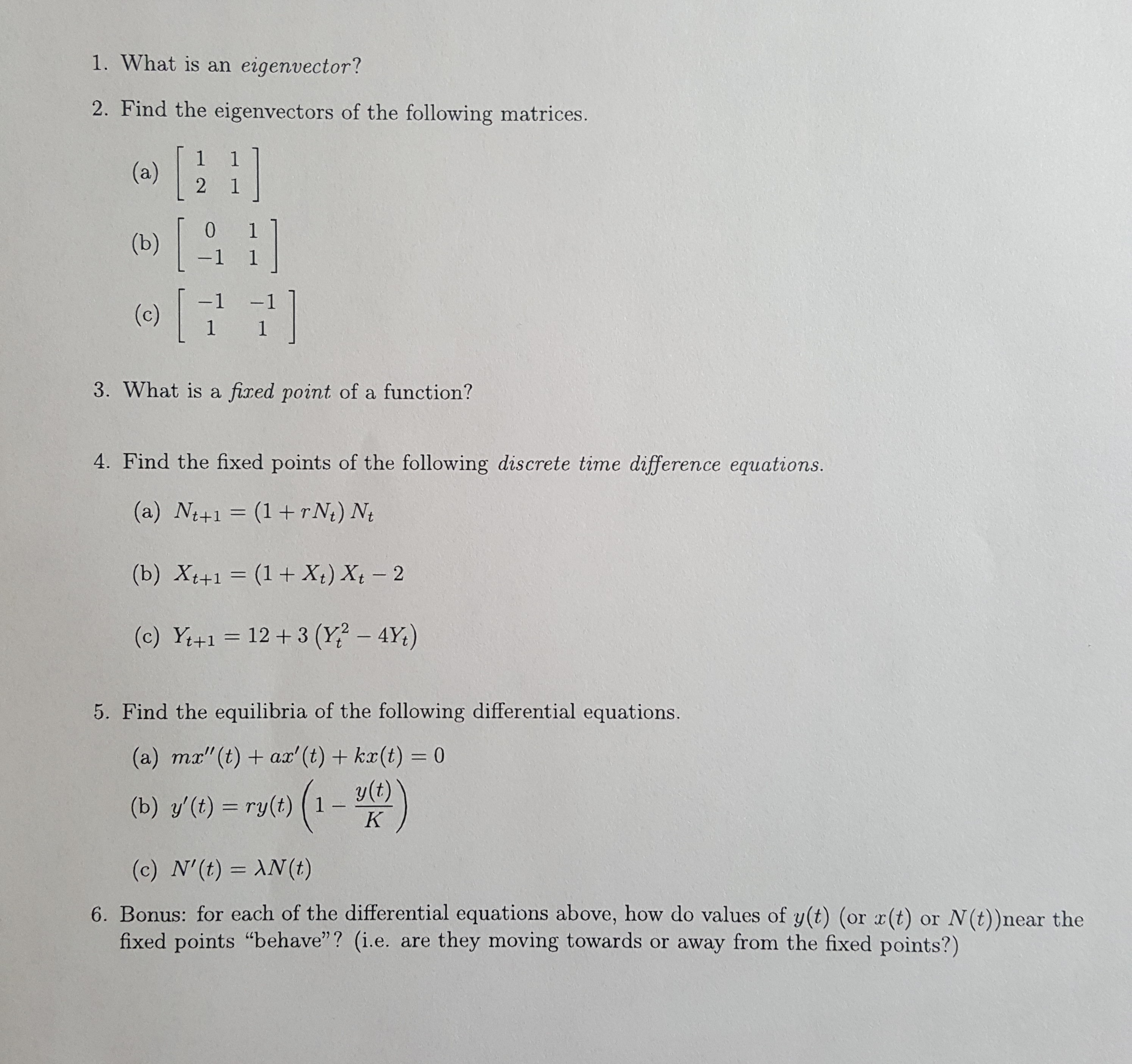# 1. What is an eigenvector?2. Find the eigenvectors of the following matrices.(a)21[0(b)-1 1-1(c)1 13. What is a fixed point of a function?4. Find the fixed points of the following discrete time difference equations.(a) Nt+1 (1+rN) Nt(b) Xt+1 (1 + X) Xt 212+3 (Y? - 4Y)(c) Y+15. Find the equilibria of the following differential equations.(a) mx"(t) ax' (t)+ kx(t) = 0y(t)1(b) y'(t) ry(t)K(c) N'(t) AN (t)6. Bonus: for each of the differential equations above, how do values of y(t) (or x(t)fixed points "behave"? (i.e. are they moving towards or away from the fixed points?)or N(t))near the

Question
36 views

I need help for problem 5c. Thanks!help_outlineImage Transcriptionclose1. What is an eigenvector? 2. Find the eigenvectors of the following matrices. (a) 2 1 [ 0 (b) -1 1 -1 (c) 1 1 3. What is a fixed point of a function? 4. Find the fixed points of the following discrete time difference equations. (a) Nt+1 (1+rN) Nt (b) Xt+1 (1 + X) Xt 2 12+3 (Y? - 4Y) (c) Y+1 5. Find the equilibria of the following differential equations. (a) mx"(t) ax' (t)+ kx(t) = 0 y(t) 1 (b) y'(t) ry(t) K (c) N'(t) AN (t) 6. Bonus: for each of the differential equations above, how do values of y(t) (or x(t) fixed points "behave"? (i.e. are they moving towards or away from the fixed points?) or N(t))near the fullscreen
check_circle

Step 1

(c)

Consider the given differential equation:

Step 2

Now, it is known that the equilibria of a differential equation is eval...

### Want to see the full answer?

See Solution

#### Want to see this answer and more?

Solutions are written by subject experts who are available 24/7. Questions are typically answered within 1 hour.*

See Solution
*Response times may vary by subject and question.
Tagged in

### Other